• Introduction
• What is in this manual
• What is Caspoc
• User interface
• Introduction
• Starting
• Simulation
• Editing
• Viewing and printing
• Getting Started
• Basic editing
• Simulation in the time domain
• Basic User Interface Topics
• Editing
• Simulation
• Viewing
• Library
• Reports
• Project management
• Circuit and Block Diagram Components
• Introduction
• Cscript and user defined functions
• Component parameters
• Modeling Topics
• Introduction
• Power Electronics
• Semiconductors
• Electrical Machines
• Electrical drives
• Power Systems
• Mechanical Systems
• Thermal Systems
• Magnetic Circuits
• Green Energy
• Coupling to FEM
• Experimenter
• Analog hardware description language
• Embedded C code Export
• Coupling to Spice
• Small Signal Analysis
• Matlab coupling
• Tips and tricks
• Appendices

## Animation of Power Electronics and Electrical Drives.

Introduction

Simulation starts to become an accepted tool for the design of power electronics and electrical drives. Over the last 30 years there have been remarkable advances in tools for the simulation of power electronics and electrical drives. Not only advances in the user interface of the programs, but also advances in the underlying methods on which these simulation tools are based.

For power electronics and motion control industries, various modeling and simulation packages are available. The integration of modeling and simulation for both motion control and power electronics into one package is not so wide spread.

In this chapter a new multilevel simulation/animation tool Caspoc®, [Simulation Research, 2001] is described, which during simulation animates the power electronic circuit. The user sees the level of the node-voltages, the level of the branch currents and most important, he can see the current path in the circuit. The simulation tool can animate any power electronic circuit or electrical drive.

In section 2, an introduction is given in the mathematical methods, which are used in simulation programs for Power Electronics and Electrical Drives. The importance of simulation for education is briefly described in section 3. A short discussion on modeling of components is presented in section 4. In section 5 the visualization of simulation results is discussed. The fact that animation is not just a toy is described in section 6 and the impact of the animation on the simulation speed is discussed in section 7. The examples in this paper will focus on the animation of power electronic circuits and electrical drives. The user has the possibility to define his own motion control models in a high-level programming or a block-diagram, enabling him to model, for example, a field oriented controller or controls for application specific drives, such as fans, pumps and automotive applications. Various examples of animation, for example the Vienna-Rectifier, conclude this paper in section 8 to 10, Modeling and Simulation methods

For the modeling of electric circuits and dynamic systems, there are generally three methods.

The State-Space approach, [Schwarz, 1987] is used in Block-diagram oriented programs, such as Simulink. The advantages of the state-space model are simplicity and simulation speed. The disadvantage is the difficulty to derive the state space equations from a circuit.

The Modified Nodal Analysis (MNA) method, [Ho, 1975], is used in Spice-based programs. The advantage is the easy mathematical model generation from a circuit. The disadvantages are the convergence problems caused by semiconductor models.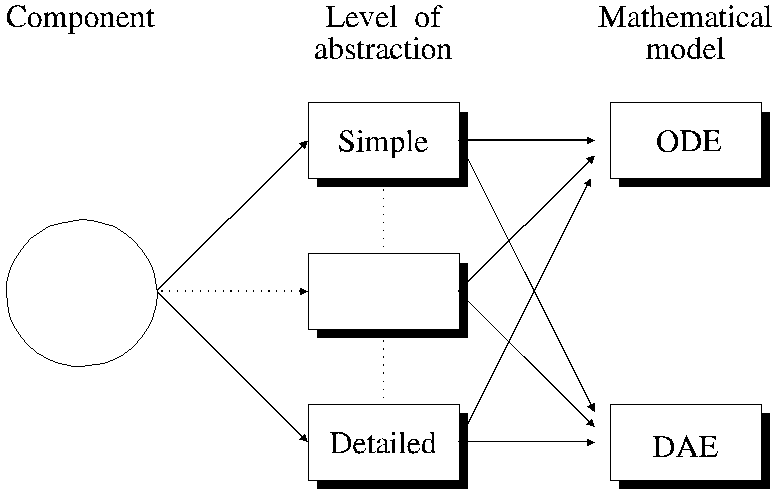Figure 1 : Complexity of models.

The modeling of a component in a Power Conversion System (PCS) is not unique. Depending on the need of the user, a model can be either simple, detailed, or can contain just enough details to model the time-domain behavior satisfactory.

The complexity of the mathematical model is not necessarily related to the complexity of the model of the component. As shown in figure 1, a simple model can contain a (non-linear) acausal mathematical relation and therefore a Differential Algebraic Equation DAE, [Schwarz, 1987], has to be used for the mathematical model:

Equation(1):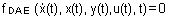The DAE describes the non-linear, possible acausal, relations among the time-varying state variables x(t), their time-derivative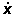(t), the variables y(t) and the input variables u(t) of a PCS.

On the other side, if a more detailed model doesn't contain any acausal relations, it can be described by a Ordinary Differential Equation ODE:

Equation(2):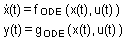Equation (2) is used in Block-diagram programs such as Simulink.

The SPICE® program is based on the MNA method. Suppose a model can be formulated as:

Equation(3):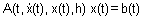The parameters of the matrix A are dependent on the variables and state variables in the vector x(t). Vector b(t) stores the values of the independent sources. The time derivative of x(t) is replaced by a numerical integration approximation, where the parameter h is the step size of the numerical integration

Multilevel modeling [Franz, 1990], [Duijsen, 1994] is a technique where different mathematical model descriptions, such as DAE, MNA and ODE, can be combined. A multilevel simulation is based on the total mathematical model of the multilevel model. A multilevel model can contain different types of models. The multilevel modeling technique is advantageous compared to other modeling techniques for two major reasons.

• Efficient modeling, because each part of the implementation like the power electronic circuit, the load or the control is described by its most efficient modeling technique. This allows a straightforward description without, for example, modeling an inverter in a block diagram or modeling a control algorithm by lumped circuit elements.
• The simulation time can be decreased, because each part of the implementation can be solved more efficiently.
Modeling and simulation for education

Modeling and simulation is a valuable tool in the area of education and training. Especially in the field of power electronics and drives, simulation is a more save method to teach. Faults and mistakes made during a simulation cause no damages or risks. To be more precisely, you can learn much more when you can simulate critical events, which are impossible to perform in reality. Take for example the maximum current through an expensive GTO. Destroying a GTO in a simulation is harmless compared to a real destruction of a GTO. Performing only simulations implies no hazardous damages to the surrounding, no replacements, no soldering, no stock of expensive GTOs.

Important for education is the availability of a library with examples. Nearly all large vendors provide examples for their simulation tools. For Spice® and Simulink® tutorials and libraries with examples for power electronics and drives can bought from third-party vendors. For Caspoc® a tutorial especially with power electronics and drive examples is available.

Simulation speed is even for education an important factor. However simulation examples can be structured such that a short simulation time is achieved by either simplifying the example or generalized modeling. Simulation speeds achieved with Spice® and Simulink® are satisfactory, with Caspoc® shorter simulation times can be achieved because of the multilevel modeling. Also of importance is the convergence of the simulation. Here Spice® has a bad performance, which can lead to a struggle with the model. In many cases the effort to make a Spice® simulation, goes mainly to including tricks in the model to enhance convergence. This leads to a waste of time for education, because students should learn how to build models, not to prevent convergence failures. Simulink® and Caspoc® have no convergence problems as known with Spice®.

Models

For the simulation of Power Electronics basic models are required, which do not exist in standard simulation programs, such as the early Spice-based programs. Recent Spice-based simulation programs have a library of sub-circuits for Power Electronic components such as the SCR, GTO, Mosfet, IGBT and, for example, electrical machines.

The complexity of the model is a very important aspect in modeling. For most simulations a basic switching behavior is satisfactory. For example, the basic switching behavior of a diode, SCR or GTO, is in many cases satisfactory to model a three-phase rectifier. A linear inductance modeled at the input is enough to simulate the commutation between the diodes in a rectifier. The produced harmonics are basically only depending on the line impedance of the input of the rectifier.

For calculating the efficiency of a SMPS, also the energy that is required to control the Mosfet is important. Here the non-linear gate capacitance has a large influence on the efficiency of the SMPS. In this case a model where the gate charge of a Mosfet is modeled is required. Multilevel modeling simplifies the modeling process for these various components.

Visualizing simulation results.

The early simulation programs produced a long list with numerical results. Nowadays most simulation programs offer the presentation of simulation results in graphical windows. The user has the ability to examine these graphical results by using a mouse, to obtain the numerical value at each point in time.

The next step is the visualization of the simulation. Most important in power electronics is the current-path. For example, freewheeling of diodes becomes clear, when the user sees the current-path changing from switches to freewheeling diodes.

The following visualization guidelines are used:

• The intensity of the voltage, current or signal is given by the color, which varies from black to red, as defined by a rainbow of colors.
• The color of a node in the electric circuit is dependent on the voltage level of that node.
• The color of an electric circuit component is dependent on the level of the current through that component.
• The color of an electric circuit wire is dependent on the level of the current through that wire.
• The color of a wire in the block-diagram is dependent on the level of the signal on that wire.
• Certain components animate depending on an event, taking place in the component, such as opening or closing of switches.

Importance of animation.

Animation of electric circuit may look like a toy. This is however absolutely wrong. Animation is a viable tool for teaching, gaining insight, checking the behavior, or searching for failure modes.

The advantages for teaching are clear. Students, for example, can see the current-paths in rectifiers or understand the freewheeling and discontinuous mode in SMPS.

For complex topologies, such as the Vienna-Rectifier [Kolar, 1994], animation can be very helpful to understand and verify the principle of the converter. During animation, it becomes clear to the user how the converter is behaving.

During animation, failure modes are detected. Even those failure modes, the user was not aware of. During failure analysis without animation it requires a lot of time to check each component. With animation, each failure is directly displayed, for example a switch is opened or closed at a wrong interval. Or some voltage levels are too high.

Practical implementation.

Animation costs time. A simulation should be as fast as possible. Animation slows down the simulation. Therefore the user should have the ability to turn the animation on or off.

If a circuit is animated, the simulation in most cases has to be slowed down, in order to follow the behavior of the system. Therefore the time-consumption of animation is in many cases not a problem.

In order to speed up the animation it is not always necessary to show each simulation step. For example, if a small time step is required for the simulation but the animation is slow varying, compared to the time step, not each time step has to be displayed. This can be achieved by not displaying each time step in the animation. Figure 2 shows a typical dialog box for animation properties.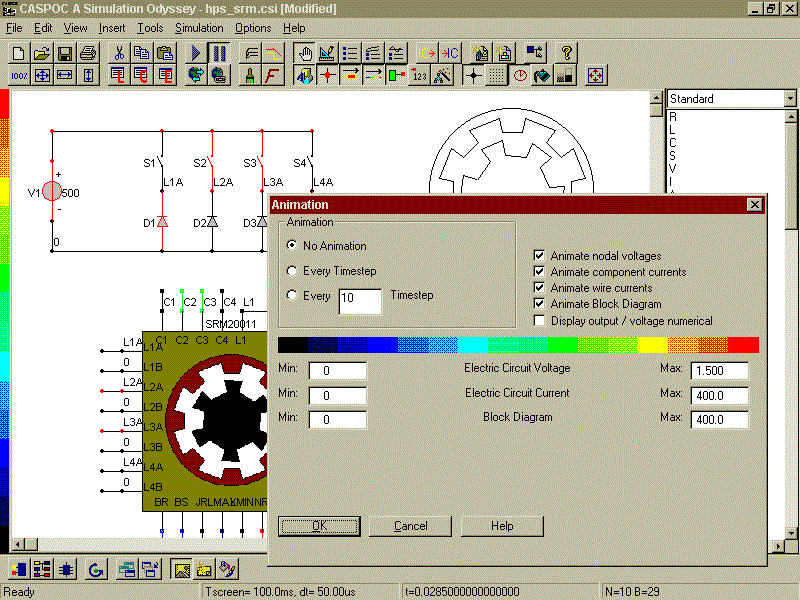Figure 2: Animation properties.

To visualize the values of voltage and current, two different levels are required. For example a SMPS operating at the AC-mains can have current levels of only up to 1 Ampere. Also the control signals can vary in value from the voltage and current level. Typical control signals can range between 0 and 1, where the on-status of a control signal is clearly signaled by a red color and the off-status is signaled by a black color.

To prevent that the schematic starts blinking like a Christmas tree, the user should have the possibility to turn on or off various animation effects. For example, the constant display of numerical values at each node can make the schematic very crowded and therefore the user should have the possibility to turn it off.

Example Vienna-Rectifier

In figure 3 the Vienna-Rectifier [Kolar, 1994] is displayed. The current-path, which is colored during the animation, is shown thick in this figure. One can see clearly that the complexity of the current-path gives valuable information on the functioning of the converter.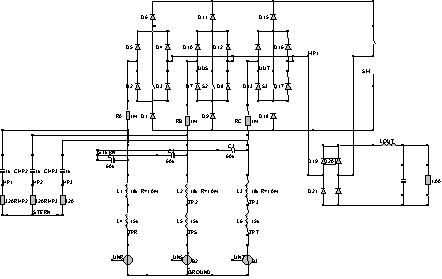Figure 3: Current-path in the Vienna-Rectifier.
Example Buck converter

In figure 4 the animation of a buck converter is shown. In the figure the freewheeling of the diode is shown. The level of the output voltage and the level of the current through the inductance L1 is displayed by two analog meters, which during the animation show the actual value.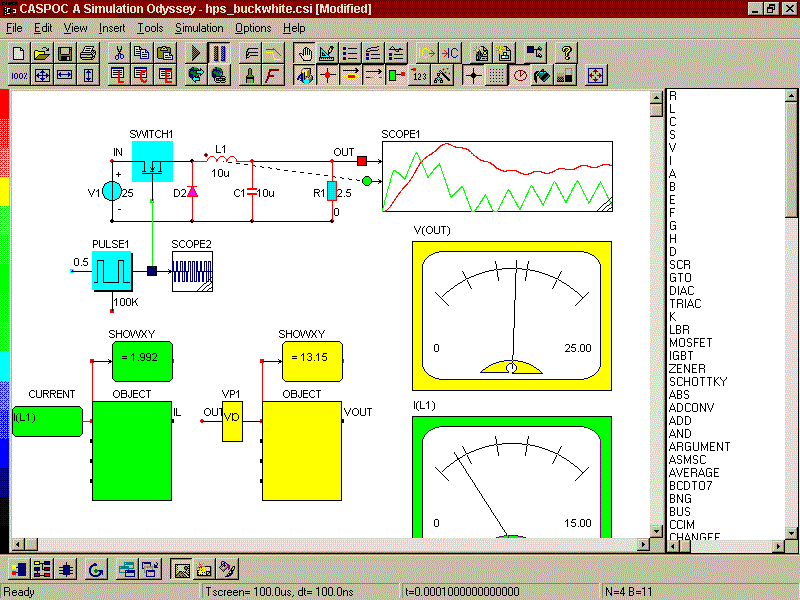Figure 4: Buck converter with analog meters.
Example DC shunt machine with crane

In figure 5 the animation of a DC shunt machine with crane is shown. The DC shunt machine is controlled by a controlled voltage source, which is regulated by a library-block 'Crane Control'. Also a controlled rectifier could have been used here. The library-block modeling the crane includes an object-block, which models the visualization of the crane. Depending on the angle of the axis of the DC shunt machine, the load is lifted by the crane.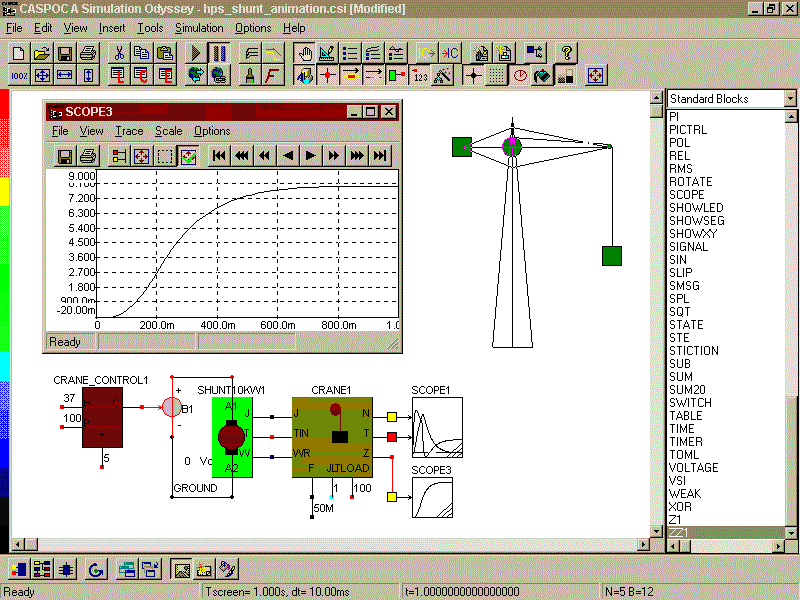Figure 5: DC shunt machine with crane.
Conclusions

Animation gives valuable information about the simulation of Power Electronics and Electrical Drives. Displaying current-paths gives insight in the behavior of the circuit and can reveal failure modes. It reveals more insight in the circuit operation then only displaying simulation results in graphs. If animation is based on simulation, also complex circuits can be animated. This makes animation a practical tool for designing power electronics and electrical drives.

References
1. Ho C-W, Ruehli A.E., Brennan P.A., The modified nodal approach to network analysis, IEEE Transactions on Circuits and Systems, Vol CAS-22, No 6, pp. 504-509, june 1975.
2. Schwarz A.F., Computer-aided design of microelectronic circuits and systems, Vol 1, Academic press 1987.
3. Franz G.A., Multilevel simulation tools for power converters, IEEE APEC Cb853-0/90/0000-0629, 1990.
4. Duijsen P.J. van, Multilevel modeling and simulation of power electronic converters and drive systems, Proceedings Power Conversion and Intelligent Motion (PCIM), 1994.
5. Kolar J.W., and Zach, F.C., A Novel Three-Phase Three-Switch Three-Level PWM Rectifier. Proceedings of the 28th Power Conversion Conference, Nuremberg, June 28-30, pp. 125-138 (1994).
6. Simulation Research, Caspoc 2001; A simulation Odyssey, www.caspoc.com , 2001